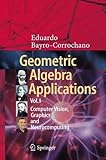# Geometric Algebra Applications Vol. I Computer Vision, Graphics and NeurocomputingGeometric Algebra Applications Vol. I: Computer Vision, Graphics and Neurocomputing
By 作者: Eduardo Bayro-Corrochano
ISBN-10 书号: 3319748289
ISBN-13 书号: 9783319748283
Edition 版本: 1st ed. 2019
Release Finelybook 出版日期: 2018-08-13
Pages 页数: 742
The Book Description robot was collected from Amazon and arranged by Finelybook
The goal of the Volume I Geometric Algebra for Computer Vision, Graphics and Neural Computing is to present a unified mathematical treatment of diverse problems in the general domain of artificial intelligence and associated fields using Clifford, or geometric, algebra.
Geometric algebra provides a rich and general mathematical framework for Geometric Cybernetics in order to develop solutions, concepts and computer algorithms without losing geometric insight of the problem in question. Current mathematical subjects can be treated in an unified manner without abandoning the mathematical system of geometric algebra for instance: multilinear algebra, projective and affine geometry, calculus on manifolds, Riemann geometry, the representation of Lie algebras and Lie groups using bivector algebras and conformal geometry.
By treating a wide spectrum of problems in a common language, this Volume I offers both new insights and new solutions that should be useful to scientists, and engineers working in different areas related with the development and building of intelligent machines. Each chapter is written in accessible terms accompanied by numerous examples, figures and a complementary appendix on Clifford algebras, all to clarify the theory and the crucial aspects of the application of geometric algebra to problems in graphics engineering, image processing, pattern recognition, computer vision, machine learning, neural computing and cognitive systems.
1 Geometric Algebra for the Twenty-First Century Cybernetics
Part I Fundamentals of Geometric Algebra
2Introduction to Geometric Algebra
3 Differentiation,Linear,and Multilinear Functions in Geometric
Algebra
4 Geometric Calculus
5 Lie Algebras,Lie Groups,and Algebra of Incidence
Part ll Euclidean,Pseudo-Euclidean Geometric Algebras,
Incidence Algebra,Conformal and Projective Geometric
Algebras
62D,3D,and 4D Geometric Algebras
7 Kinematics of the 2D and 3D Spaces
8 Conformal Geometric Algebra
9 The Geometric Algebras G6,0,2+,G6,3,G9,3+,G6,0,6+
10 Programming Issues
Part ll Image Processing and Computer Vision
11 Quaternion-Clifford Fourier and Wavelet Transforms
12 Geometric Algebra of Computer Vision
Part IV Machine Learning
13 Geometric Neurocomputing
Part V Applications of GA in lmage Processing,Graphics and
Computer Vision
14 Applications of Lie Filters,Quaternion Fourier,and Wavelet
Transforms
15 Invariants Theory in Computer Vision and Omnidirectional
Vision
16 Geometric Algebra Tensor Voting,Hough Transform,Voting
and Perception Using Conformal Geometric Algebra
17 Modeling and Registration of Medical Data
Part VI Applications of GA in Machine Learning
18 Applications in Neurocomputing
19 Neurocomputing for 2D Contour and 3D Surface
Reconstruction
20 Clifford Algebras and Related Algebras

### 觉得文章有用就打赏一下文章作者

#### 支付宝扫一扫打赏#### 微信扫一扫打赏• QQ咨询
• 回顶